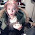Friday, March 1, 2013

Milestone presents!

5000 pageviews for me just ranting is pretty cool, so have some surprise processing code!  I won't tell you what it does, basically it came out of a Unity UI experiment Annie's been working on and my desire to learn how processing's PVector interface really works.  Found some interesting quirks, tho having more to do with 2d and 3d and PVector being a 3d construct vs PVector's implementation, i'm going to say (since Shiffman's a genius and probably knows what he's doing).  Anyway...have fun (and send Annie a hey if you end up using this code for something)!  If it's any hint, i call this "Spider Silk"...(poetic, no?)

```float thresh = 0.5;
//world vectors
PVector v_ctr_w = new PVector(0,0);
PVector v_0_w = new PVector(0,0);
PVector v_1_w = new PVector(0,0);

//image vectors
PVector v_ctr_i = new PVector(0,0);
PVector v_0_i = new PVector(0,0);
PVector v_1_i = new PVector(0,0);

void setup()
{
size(500,500,P2D);
stroke(128);
}

void draw()
{
background(0);
v_ctr_i.set(map(v_ctr_w.x,-1,1,0,width),map(v_ctr_w.y,-1,1,0,height),0);
v_0_i.set(mouseX,mouseY,0);
v_0_w.set(map(v_0_i.x,0,width,-1,1),map(v_0_i.y,0,height,-1,1),0);

line(v_ctr_i.x,v_ctr_i.y,v_0_i.x,v_0_i.y);
ellipse(v_ctr_i.x,v_ctr_i.y,50,50);
fill(0);
ellipse(v_0_i.x,v_0_i.y,30,30);

fill(255);
if(v_0_w.mag()<thresh)
v_1_w.set(v_0_w.x,v_0_w.y,0);
else
v_1_w = PVector.mult(v_0_w,thresh/v_0_w.mag());

v_1_i.set(map(v_1_w.x,-1,1,0,width),map(v_1_w.y,-1,1,0,height),0);
ellipse(v_1_i.x,v_1_i.y,15,15);
}

void mousePressed()
{
thresh = v_0_w.mag();
}
```

1 comment:

1.m-u-a-ha-ha-ha-ha